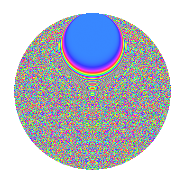# Properties

 Label 1003.2.rLevel 1003 Weight 2 Character orbit r Rep. character $$\chi_{1003}(9,\cdot)$$ Character field $$\Q(\zeta_{232})$$ Dimension 9856 Sturm bound 180

# Related objects

## Defining parameters

 Level: $$N$$ = $$1003 = 17 \cdot 59$$ Weight: $$k$$ = $$2$$ Character orbit: $$[\chi]$$ = 1003.r (of order $$232$$ and degree $$112$$) Character conductor: $$\operatorname{cond}(\chi)$$ = $$1003$$ Character field: $$\Q(\zeta_{232})$$ Sturm bound: $$180$$

## Dimensions

The following table gives the dimensions of various subspaces of $$M_{2}(1003, [\chi])$$.

Total New Old
Modular forms 10304 10304 0
Cusp forms 9856 9856 0
Eisenstein series 448 448 0

## Trace form

 $$9856q - 108q^{2} - 108q^{3} - 108q^{5} - 108q^{6} - 108q^{7} - 124q^{8} - 132q^{9} + O(q^{10})$$ $$9856q - 108q^{2} - 108q^{3} - 108q^{5} - 108q^{6} - 108q^{7} - 124q^{8} - 132q^{9} - 108q^{10} - 116q^{11} - 108q^{12} - 124q^{14} - 96q^{15} + 112q^{16} - 116q^{17} - 240q^{18} - 100q^{19} - 140q^{20} - 92q^{22} - 124q^{23} - 156q^{24} - 108q^{25} - 92q^{26} - 84q^{27} - 140q^{28} - 116q^{29} - 76q^{31} - 164q^{32} - 184q^{33} - 140q^{34} - 184q^{35} - 180q^{36} - 84q^{37} - 132q^{39} - 132q^{40} - 100q^{41} - 196q^{42} - 116q^{43} - 140q^{44} - 88q^{45} - 76q^{46} - 140q^{48} - 92q^{49} + 272q^{50} - 188q^{51} - 328q^{52} - 116q^{53} - 848q^{54} - 20q^{56} - 68q^{57} - 232q^{58} - 116q^{59} + 1176q^{60} - 60q^{61} - 108q^{62} - 108q^{63} - 180q^{65} - 116q^{66} + 32q^{67} - 180q^{68} - 280q^{69} - 156q^{70} - 356q^{71} - 140q^{73} - 60q^{74} - 84q^{75} - 252q^{76} - 52q^{77} - 52q^{78} - 108q^{79} - 108q^{80} - 188q^{82} - 180q^{83} - 200q^{84} - 60q^{85} - 280q^{86} - 148q^{87} - 148q^{88} - 92q^{90} - 196q^{91} - 252q^{92} - 12q^{93} - 52q^{94} - 108q^{95} - 92q^{96} - 164q^{97} + 312q^{99} + O(q^{100})$$

## Decomposition of $$S_{2}^{\mathrm{new}}(1003, [\chi])$$ into newform subspaces

The newforms in this space have not yet been added to the LMFDB.

## Hecke Characteristic Polynomials

There are no characteristic polynomials of Hecke operators in the database